Test: Double Sideband Suppressed Carrier (DSB-SC) Modulation

# Test: Double Sideband Suppressed Carrier (DSB-SC) Modulation - Electronics and Communication Engineering (ECE)

Test Description

## 10 Questions MCQ Test GATE ECE (Electronics) 2023 Mock Test Series - Test: Double Sideband Suppressed Carrier (DSB-SC) Modulation

Test: Double Sideband Suppressed Carrier (DSB-SC) Modulation for Electronics and Communication Engineering (ECE) 2023 is part of GATE ECE (Electronics) 2023 Mock Test Series preparation. The Test: Double Sideband Suppressed Carrier (DSB-SC) Modulation questions and answers have been prepared according to the Electronics and Communication Engineering (ECE) exam syllabus.The Test: Double Sideband Suppressed Carrier (DSB-SC) Modulation MCQs are made for Electronics and Communication Engineering (ECE) 2023 Exam. Find important definitions, questions, notes, meanings, examples, exercises, MCQs and online tests for Test: Double Sideband Suppressed Carrier (DSB-SC) Modulation below.
Solutions of Test: Double Sideband Suppressed Carrier (DSB-SC) Modulation questions in English are available as part of our GATE ECE (Electronics) 2023 Mock Test Series for Electronics and Communication Engineering (ECE) & Test: Double Sideband Suppressed Carrier (DSB-SC) Modulation solutions in Hindi for GATE ECE (Electronics) 2023 Mock Test Series course. Download more important topics, notes, lectures and mock test series for Electronics and Communication Engineering (ECE) Exam by signing up for free. Attempt Test: Double Sideband Suppressed Carrier (DSB-SC) Modulation | 10 questions in 30 minutes | Mock test for Electronics and Communication Engineering (ECE) preparation | Free important questions MCQ to study GATE ECE (Electronics) 2023 Mock Test Series for Electronics and Communication Engineering (ECE) Exam | Download free PDF with solutions
 1 Crore+ students have signed up on EduRev. Have you?
Test: Double Sideband Suppressed Carrier (DSB-SC) Modulation - Question 1

### What is the power transmitted (in W) by a DSB-SC signal when the carrier wave power is 80 W and modulation index is 0.4?

Detailed Solution for Test: Double Sideband Suppressed Carrier (DSB-SC) Modulation - Question 1

Concept:

The total transmitted power for an AM system is given by: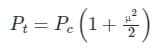P= Carrier Power

μ = Modulation Index

Since the given modulation is DSB-SC, the carrier is suppressed.
∴ The transmitted power will be: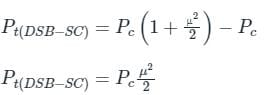Calculation:

Given

μ = 0.4

Pc = 80 W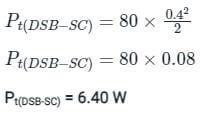Test: Double Sideband Suppressed Carrier (DSB-SC) Modulation - Question 2

### An AM broadcast station is found to be transmitting modulating frequencies up to 5 kHz and the station is allowed to transmit on a frequency of 950 kHz, then what will be the bandwidth (in kHz) allotted to the station?

Detailed Solution for Test: Double Sideband Suppressed Carrier (DSB-SC) Modulation - Question 2

Concept:

Standard expression for AM expression for a message signal AmCosωmt is

S(t) = AC(1 + μCosωmt )cos ωct

Where;

ωm → Modulating frequency (rad/sec) = 2πfm

Bandwidth = 2fm

Calculation:

Given;

Modulating frequency (fm) = 5 KHz

Bandwidth = 2fm = (2 × 5) = 10 KHz

Test: Double Sideband Suppressed Carrier (DSB-SC) Modulation - Question 3

### Which of the following equation represents the full-carrier AM (DSB-full carrier)?

Detailed Solution for Test: Double Sideband Suppressed Carrier (DSB-SC) Modulation - Question 3

Concept:

The expression of the full-carrier AM (DSB-full-carrier) = (Ec + m(t)) × sin(ωct)

• Ec is peak amplitude of the carrier signal i.e  Ecsin(ωct).
• ωc is the frequency of the carrier signal in rad/sec.
• m(t) is modulating or the message signal.

Calculation:

Given, m(t) = Emsin(ωmt) , where Em is the peak amplitude of the messege signal and ωm is the frequency of the message signal in rad/sec.

.Equation for full-carrier AM signal:

v(t) =  (Ec + m(t)) × sin(ωct)

⇒ v(t) =  (Ec + Emsin (ωmt) ) × sin(ωct)

Hence, the equation of the full-carrier AM (DSB-full carrier) is v(t) = (Ec + Em sin(ωmt)) × sin(ωct).

Test: Double Sideband Suppressed Carrier (DSB-SC) Modulation - Question 4

What will be the upper sideband and lower sideband frequencies for amplitude modulation of a 5-kHz signal with 40-kHz carrier frequency?

Detailed Solution for Test: Double Sideband Suppressed Carrier (DSB-SC) Modulation - Question 4

Concept:

An amplitude modulation spectrum is represented as: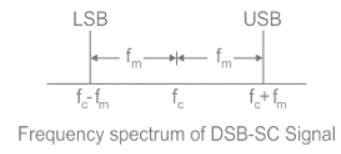fc = Carrier Frequency

fm = Signal frequency

fc + fm = Upper Sideband

fc - fm = Lower Sideband

Analysis:

Given fc = 40 kHz and fm = 5 kHz

The upper and lower sidebands after Amplitude modulation will be:

Upper Sideband = fc + fm = 40 + 5 kHz = 45 kHz

Lower Sideband = fc - fm = 40 - 5 kHz = 35 kHz

Test: Double Sideband Suppressed Carrier (DSB-SC) Modulation - Question 5

A type of amplitude modulation in which carrier is suppressed is called

Detailed Solution for Test: Double Sideband Suppressed Carrier (DSB-SC) Modulation - Question 5

In a balanced modulator, 2 AM-modulators are connected in a way that the resultant signal does not contain the carrier spectrum, i.e. to generate a Double Side-band suppressed carrier (DSB-SC).

Analysis:

The circuit diagram of a balanced modulator is as shown: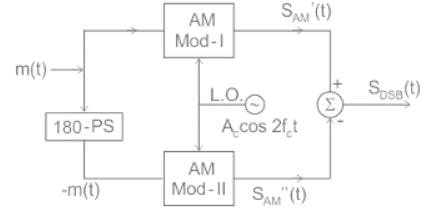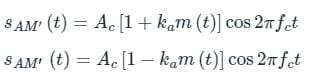The resultant DSB signal is the difference of the two, i.e.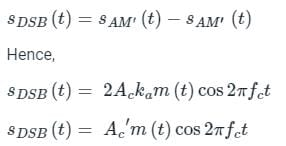The frequency spectrum of the DSB-SC signal:

The standard DSB-SC equation is given as: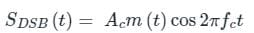Assuming a single tone message signal as: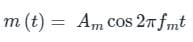The DSB-SC signal can be written as: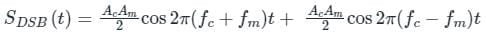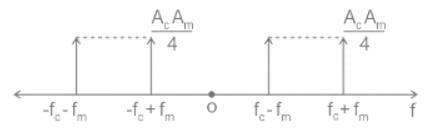∴ The output of balanced modulator contains the modulation frequency, LSB and USB.

Test: Double Sideband Suppressed Carrier (DSB-SC) Modulation - Question 6

The ring modulator is generally used for generating

Detailed Solution for Test: Double Sideband Suppressed Carrier (DSB-SC) Modulation - Question 6

The ring modulator and balanced modulator is generally used for the modulation of DSB SC signal.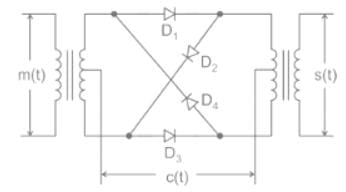SSB/SC is generated using a balanced modulator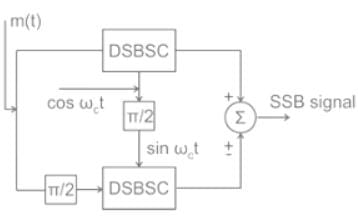Test: Double Sideband Suppressed Carrier (DSB-SC) Modulation - Question 7

Which of the following requires the least bandwidth?

Detailed Solution for Test: Double Sideband Suppressed Carrier (DSB-SC) Modulation - Question 7

DSB -SC:

1) It is a form of Amplitude modulation where only the sidebands are transmitted and the carrier is suppressed.

2) The advantage is 66% power saving compared to conventional AM.

3) The Bandwidth requirement is of 2fm, with fm being the maximum frequency component of the message signal.

4) It is used for transmitting stereo information.

Single Side Band (SSB SC):

1) SSB SC is a form of amplitude modulation where only a single sideband is transmitted and 1 sideband and carrier are suppressed.

2) The advantage of SSB is bandwidth and power-saving over both conventional AM and DSB SC, i.e. DSB SC has more power consumption than SSB SC.

3) The Bandwidth requirement is only fm, ∴ It needs half the bandwidth than the DSB-SC transmission.

Comparison: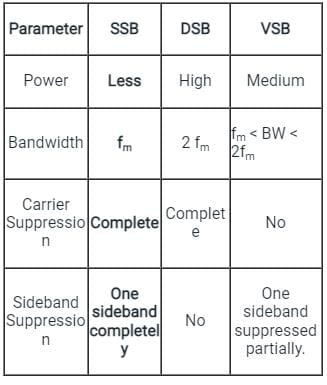Test: Double Sideband Suppressed Carrier (DSB-SC) Modulation - Question 8

For the modulated signal x(t) = m(t) cos (2πfct), the message signal m(t) = 4 cos (1000πt) and the carrier frequency fc is 1 MHz. The signal x(t) is passed through a demodulator, as shown in the figure below. The output y(t) of the demodulator is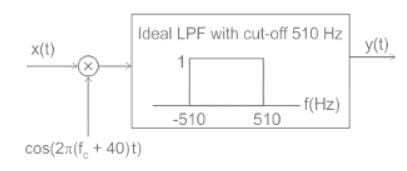Detailed Solution for Test: Double Sideband Suppressed Carrier (DSB-SC) Modulation - Question 8

Concept: Low pass filter allow the low frequency components ( up to Bandwidth of filter) to pass through it.

Calculation:

x(t) = m(t) cos (2πfct)

m(t) = 4 cos (1000 πt)

The output of the multiplier(s(t)) will be:

s(t) = x(t) cos (2π(fc + 40)t)

s(t) = m(t) cos (2πfct) cos (2π(fc + 40)t)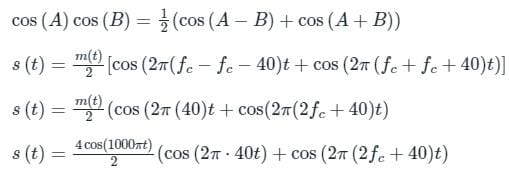s(t) = 2 cos (100 πt)⋅cos (2π 40t) + 2 cos (1000 πt) cos (2π(2fc + 40)t

Again using the same trigonometric identify, the above equation can be written as:

s(t) = cos (1000πt – 80πt) + cos (1000πt + 80πt) + cos (1000πt – 2π(2fc + 40)t) + cos (1000πt + 2π (2fc + 40)t)

s(t) = cos (920πt) + cos (1080πt) + cos (1000πt – 2π (2fc - 40)t + cos(1000πt + 2π (2fc + 40)t)

The frequency specimen of s(t) can be drawn as: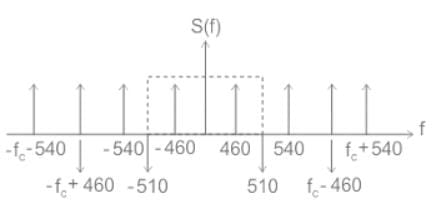The output of the low pass filter will contain frequencies of 460 Hz only as shown: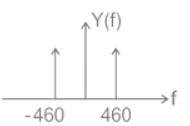∴ y(t) = cos (920 πt)

Test: Double Sideband Suppressed Carrier (DSB-SC) Modulation - Question 9

A DSB-SC signal is generated using the carrier signal cos (ωct + θ) and modulating signal m(t). What is the envelope detector output of this DSB-SC signal?

Detailed Solution for Test: Double Sideband Suppressed Carrier (DSB-SC) Modulation - Question 9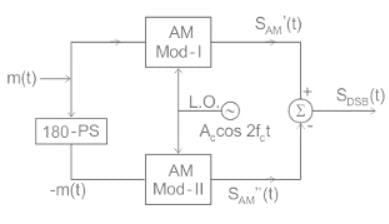s1(t) = Ac [1 + kam(t) ] cosωct

s2(t) = Ac [1 - kam(t) ] cosωct

s(t) =  Ac [1 + kam(t) ] cosωct -  Ac [ 1 - kam(t) ] cosωct

= 2 Ac ka m(t) cosωct

s(t) ∝ m(t) cosωct

Hence, Envelope of s(t) = |m(t)|

Test: Double Sideband Suppressed Carrier (DSB-SC) Modulation - Question 10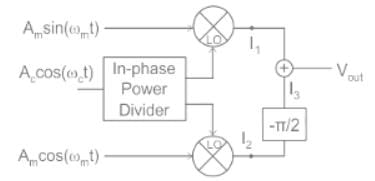In this circuit, Vout is:

Detailed Solution for Test: Double Sideband Suppressed Carrier (DSB-SC) Modulation - Question 10

Concept:

A single sideband (SSB) AM Signal is represented as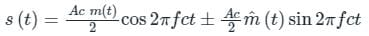m̂(t) → represent Hilbert transform of message signal

(-) → sign represent USB

(+) → sign represent LSB

Hilbert transform:

It is a specific Linear operator that takes a function u(t) of a real variable and produces another function of real variable H(u)(t)

→ It imparts a phase shift of ± 90° (π/2 radians) to every frequency component of a function.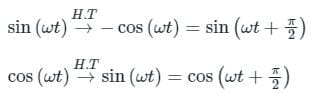Explanation:

From the given Block diagram.

I1 = (Am sin ωmt)(Ac cos ωct)

I2 = (Am cos ωmt)(Ac cos ωct)

Vout = I1 + I3

From I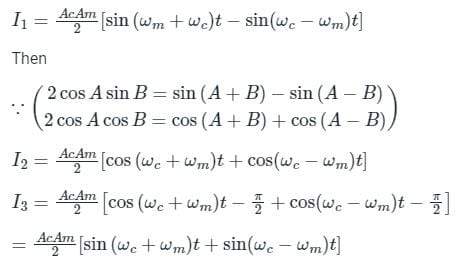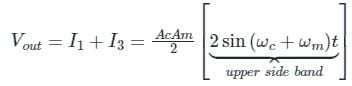Option (A) correct choice.

Short trick:

Observe the Vout in block diagram i.e. Vout = I1 + I3

But in I3 there is –π/2 phase change so the SSB becomes in the Form of

S(t) = m(t) cos ωct – m̂(t) sin ωct

And (-) sign represent USB only.

So the student can tick the correct answer.

## GATE ECE (Electronics) 2023 Mock Test Series

21 docs|263 tests
Information about Test: Double Sideband Suppressed Carrier (DSB-SC) Modulation Page
In this test you can find the Exam questions for Test: Double Sideband Suppressed Carrier (DSB-SC) Modulation solved & explained in the simplest way possible. Besides giving Questions and answers for Test: Double Sideband Suppressed Carrier (DSB-SC) Modulation, EduRev gives you an ample number of Online tests for practice

## GATE ECE (Electronics) 2023 Mock Test Series

21 docs|263 tests(Scan QR code)